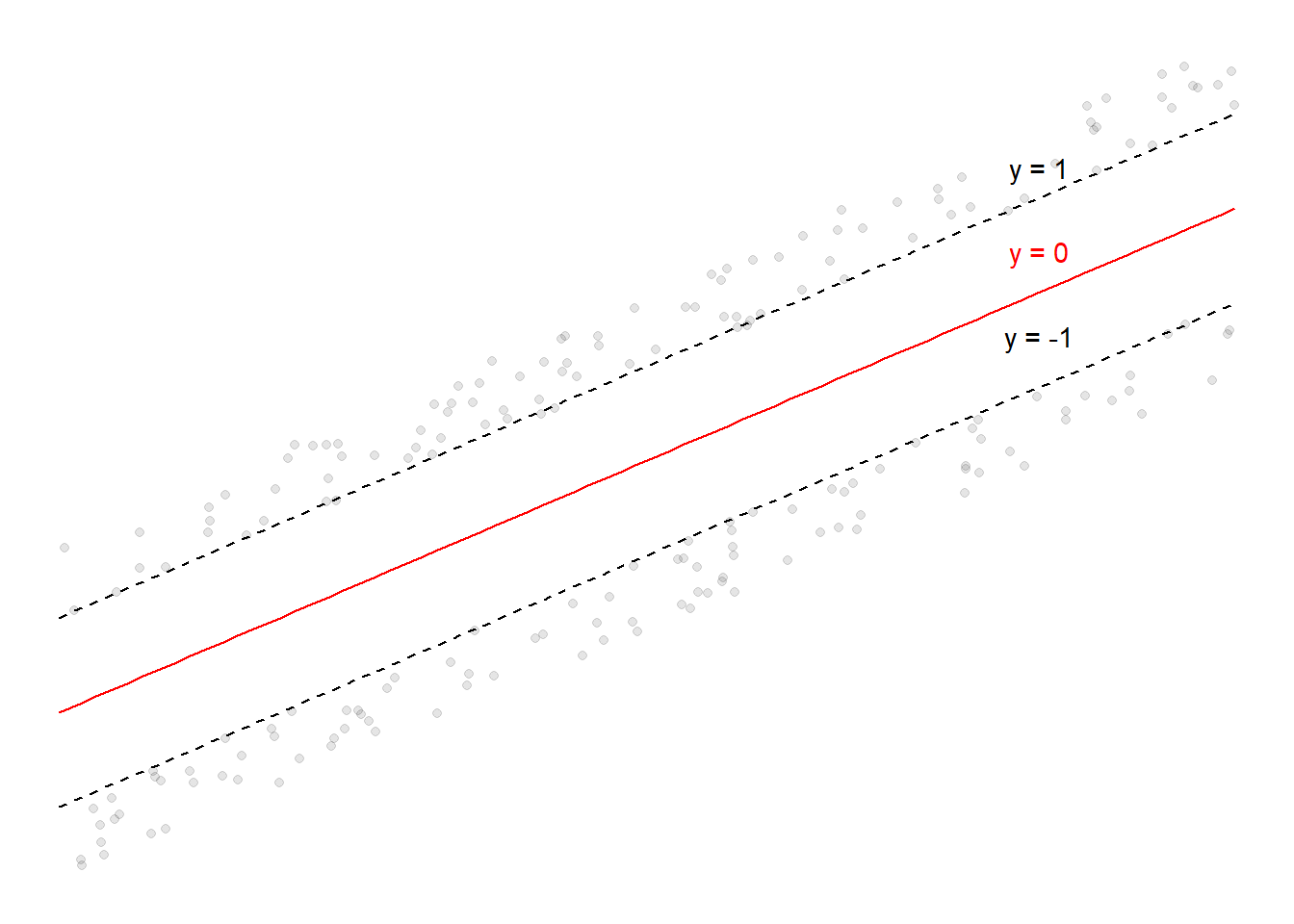# Machine Learning: The Mathematics of Support Vector Machines - Part 1

Linearly Separable Case, Formulating the Objective Function

Views and opinions expressed are solely my own.

## Introduction

The purpose of this post is to discuss the mathematics of support vector machines (SVMs) in detail, in the case of linear separability.

## Background

SVMs are a tool for classification. The idea is that we want to find two lines (linear equations) so that a given set of points are linearly separable according to a binary classifier, coded as $$\pm 1$$, assuming such lines exist. These lines are given by the black lines given below.

library(ggplot2)
set.seed(45)

plus_1 <- data.frame(x = runif(100, min = -3, max = 3))
plus_1$y <- plus_1$x + runif(100, min = 1.1, max = 2)

minus_1 <- data.frame(x = runif(100, min = -3, max = 3))
minus_1$y <- minus_1$x + runif(100, min = -2, max = -1.1)

ggplot() +
geom_point(data = plus_1,
aes(x = x, y = y),
alpha = 0.1) +
geom_point(data = minus_1,
aes(x = x, y = y),
alpha = 0.1) +
geom_text(aes(x = 2, y = 3.5, label = "y = 1"),
color = "black") +
stat_function(fun = function(x){ x + 1.142233 },
color = "black",
linetype = "dashed") +
geom_text(aes(x = 2, y = 2.5, label = "y = 0"),
color = "red") +
stat_function(fun = function(x){ x + 0.0149345 },
color = "red") +
geom_text(aes(x = 2, y = 1.5, label = "y = -1"),
color = "black") +
stat_function(fun = function(x){ x - 1.112364 },
color = "black",
linetype = "dashed") +
theme_void() +
scale_x_continuous(limits = c(-3, 3)) +
scale_y_continuous(limits = c(-5, 5))Points at or above the $$y = 1$$ line are classified as $$+1$$, and points at or below the $$y = -1$$ line are classified as $$-1$$. The line labeled “$$y = 0$$” is known as the decision boundary. The $$y = 1$$ and $$y = -1$$ lines are the lines touching the points which are closest to the decision boundary.

Assuming the lines above all have common parameter vector $$\boldsymbol\beta \in \mathbb{R}^p \setminus \{\mathbf{0}\}$$ (which we assume only includes the slope-related terms) and intercept term $$\beta_0 \in \mathbb{R}$$, we seek the decision boundary following the form of a linear equation: $$\boldsymbol\beta^{T}\mathbf{x} + \beta_0$$. At the decision boundary, we assume the classifier is $$0$$, hence we assume $$\boldsymbol\beta^{T}\mathbf{x} + \beta_0 = 0$$ at the decision boundary.

Let $$(\mathbf{x}_1, y_1), \dots, (\mathbf{x}_N, y_N)$$ denote the training data in $$\mathbb{R}^{p} \times \{-1, 1\}$$, with each $$y_i \in \{-1, +1\}$$ for each $$i = 1, \dots, N$$. Let $$\omega_{+1}$$, $$\omega_{-1}$$ denote the subsets of $$\mathbb{R}^p$$ for which the corresponding classifiers are $$+1$$ and $$-1$$ respectively. Then we assume that \begin{align*} &\boldsymbol\beta^{T}\mathbf{x}_i + \beta_0 \geq 1\text{, }\quad \mathbf{x}_i \in \omega_{+1} \\ &\boldsymbol\beta^{T}\mathbf{x}_i + \beta_0 \leq -1\text{, }\quad \mathbf{x}_i \in \omega_{-1} \end{align*} Since $$y_i \in \{-1, +1\}$$, the above two conditions are equivalent to $\begin{equation*} y_i(\boldsymbol\beta^{T}\mathbf{x}_i + \beta_0) \geq 1\text{, } \quad\mathbf{x}_i \in \mathbb{R}^{p}\text{.} \end{equation*}$ However, it is worth noting there are infinitely many possible decision boundaries that satisfy these conditions. We have to choose some sort of criteria for optimality.

## An Intermediate Result

Consider the decision boundary $$\boldsymbol\beta^{T}\mathbf{x} + \beta_0 = 0$$ and a point $$\mathbf{z} = \begin{bmatrix} z_1 & z_2 & \cdots & z_{p} \end{bmatrix}^{T}\in \mathbb{R}^{p}$$. We aim to calculate the perpendicular distance from $$\mathbf{z}$$ to $$\mathbf{x}$$, given the constraint $$\boldsymbol\beta^{T}\mathbf{x} + \beta_0 = 0$$. Consider minimizing the squared Euclidean distance from $$\mathbf{x}$$ to $$\mathbf{z}$$ subject to $$\mu(\mathbf{x}) = \boldsymbol\beta^{T}\mathbf{x} + \beta_0 = 0$$, by the method of Lagrange multipliers. We work with the function \begin{align*} L(\mathbf{x}, \lambda) = \sum_{i=1}^{p}(x_i - z_i)^2 + \lambda\left(\beta_1x_1 + \cdots + \beta_px_p + \beta_0\right)\text{.} \end{align*} Then $\begin{equation*} \dfrac{\partial L}{\partial x_i} = 2(x_i - z_i) + \lambda\beta_i = 0 \implies (x_i -z_i)^2 = \dfrac{\lambda^2\beta_i^2}{4}\text{.} \end{equation*}$ Because $$\beta_1x_1 + \cdots + \beta_px_p + \beta_0 = 0$$, we have that $\begin{equation*} \sum_{i=1}^{p}\beta_ix_i + \beta_0 = \sum_{i=1}^{p}\left(z_i\beta_i-\dfrac{\lambda\beta_i^2}{2}\right) + \beta_0 = \sum_{i=1}^{p}z_i\beta_i-\dfrac{\lambda}{2}\sum_{j=1}^{p}\beta_j^2 + \beta_0 = 0\text{.} \end{equation*}$ This implies that $\begin{equation*} \lambda = \dfrac{2}{\sum_{j=1}^{p}\beta_j^2}\left(\beta_0 + \sum_{i=1}^{p}z_i\beta_i\right) = \dfrac{2}{\|\boldsymbol\beta\|^2_2}\mu(\mathbf{z}) \implies \dfrac{\lambda^2\beta_i^2}{4} = \dfrac{[\mu(\mathbf{z})]^2\beta_i^2}{\|\boldsymbol\beta\|_2^4}\text{.} \end{equation*}$ The squared Euclidean distance is then given by $\begin{equation*} \sum_{i=1}^{p}(x_i - z_i)^2 = \dfrac{[\mu(\mathbf{z})]^2}{\|\boldsymbol\beta\|_2^4}\sum_{i=1}^{p}\beta_i^2 = \dfrac{[\mu(\mathbf{z})]^2\|\boldsymbol\beta\|_2^2}{\|\boldsymbol\beta\|_2^4} = \dfrac{[\mu(\mathbf{z})]^2}{\|\boldsymbol\beta\|_2^2} \end{equation*}$ hence the Euclidean distance from $$\mathbf{x}$$ to $$\mathbf{z}$$ is given by $\begin{equation*} \sqrt{\sum_{i=1}^{p}(x_i - z_i)^2} = \dfrac{|\mu(\mathbf{z})|}{\|\boldsymbol\beta\|_2}\text{.} \end{equation*}$

## Optimality in SVMs

Now suppose $$\mathbf{x}_{+1}$$ and $$\mathbf{x}_{-1}$$ are the closest points to the decision boundary with classifications $$+1$$ and $$-1$$ respectively. We obtain that \begin{align*} &\boldsymbol\beta^{T}\mathbf{x}_{+1} + \beta_0 = 1 \\ &\boldsymbol\beta^{T}\mathbf{x}_{-1} + \beta_0 = -1\text{.} \end{align*} Hence, the perpendicular distances of these two points to any point $$\mathbf{x}$$ on the decision boundary is given by \begin{align*} &\dfrac{|\mu(\mathbf{x}_{+1})|}{\|\boldsymbol\beta\|_2} = \dfrac{|\boldsymbol\beta^{T}\mathbf{x}_{+1} + \beta_0|}{\|\boldsymbol\beta\|_2} = \dfrac{1}{\|\boldsymbol\beta\|_2} \\ &\dfrac{|\mu(\mathbf{x}_{-1})|}{\|\boldsymbol\beta\|_2} = \dfrac{|\boldsymbol\beta^{T}\mathbf{x}_{-1} + \beta_0|}{\|\boldsymbol\beta\|_2} = \dfrac{1}{\|\boldsymbol\beta\|_2} \text{.} \end{align*} The sum of these two distances, given by $$M = \dfrac{2}{\|\boldsymbol\beta\|_2}$$, is called the margin. Geometrically, one can think of the margin as the total perpendicular distances from the decision boundary to the closest points ($$\mathbf{x}_{+1}$$, $$\mathbf{x}_{-1}$$) of each of the states of the binary classifier. These points are known as the support vectors.

The optimization problem with regard to SVMs is \begin{align*} &\max \dfrac{2}{\|\boldsymbol\beta\|_2}\\ &\text{subject to }y_i(\boldsymbol\beta^{T}\mathbf{x}_i + \beta_0) \geq 1\text{, } i = 1, \dots, n\text{.} \end{align*} However, the objective function $$\dfrac{2}{\|\boldsymbol\beta\|_2}$$ is difficult to work with; instead, we minimize the reciprocal and square the Euclidean norm to avoid having to deal with square roots, leading to the problem \begin{align*} &\min \dfrac{\|\boldsymbol\beta\|_2^2}{2}\\ &\text{subject to }y_i(\boldsymbol\beta^{T}\mathbf{x}_i + \beta_0) \geq 1\text{, } i = 1, \dots, n\text{.} \end{align*} Note that this is a convex optimization problem.

## Conclusion and Next Steps

In this post, we formulated the SVM problem as a convex optimization problem. In a future post, I will discuss more on conditions guaranteeing a solution to the SVM problem (i.e., the Karush-Kuhn-Tucker conditions).